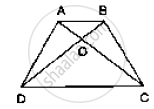# In the Below Fig. Abcd is a Trapezium in Which Ab || Dc. Prove that Ar (δAod) = Ar(δBoc). - Mathematics

In the below fig. ABCD is a trapezium in which AB || DC. Prove that ar (ΔAOD) =
ar(ΔBOC).#### Solution

Given: ABCD is a trapezium with  AB ||DC
To prove: ar (ΔAOD) = ar (BOC)
Proof:

Since ΔADC and ΔBDC are on the same base DC and between same parallels AB and DC
Then, ar (ΔADC )  = ar (ΔBDC)

⇒ ar (ΔAOD) + ar ( DOC) = ar (ΔBOC) + ar ( ΔDOC)

⇒ ar (ΔAOD) = ar (ΔBOC)

Concept: Corollary: A rectangle and a parallelogram on the same base and between the same parallels are equal in area.
Is there an error in this question or solution?

#### APPEARS IN

RD Sharma Mathematics for Class 9
Chapter 14 Areas of Parallelograms and Triangles
Exercise 14.3 | Q 7 | Page 45

Share